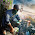# Implement Parallel Reduction using Min, Max, Sum and Average operations.

## Output:-

Elements:-
9295 2008 8678 8725 418 2377 12675 13271 4747 2307
The minimum element is 418 .
The min element (using CPU) is 418.
The maximum element is 13271.
The sum of elements is 57447.
The average of elements is 5744.
The average of elements is 5744.

#### 1 comment:

1.The sum is wrong!